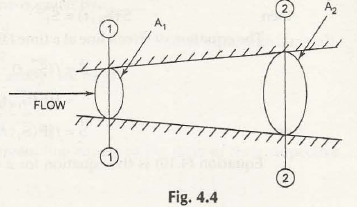Continuity equation describes the phenomenon of fluid flow through a pipe converse mass.

Let us consider the conservation of mass in closed system. So, we get the following equation-

A1V11t = A2V22t

Or,

A1V11 = A2V22

Where,

A1 ,A2 = Cross-sectional area at inlet and outlet of the given pipe

V1 ,V2 = Velocities at inlet and outlet of pipe

1 ,2 = Densities of liquid at inlet and outlet of pipe

Consider Fig. 4.4 shows flow of an incompressible fluid,In case of incompressible fluid, we know that densities are same at inlet and outlet-

Hence,

1 =2

From the above equation,

A1V1 = A2V2

Links of Next Mechanical Engineering Topics:-### Customer Reviews

My Homework Help
Rated 5.0 out of 5 based on 510 customer reviews at